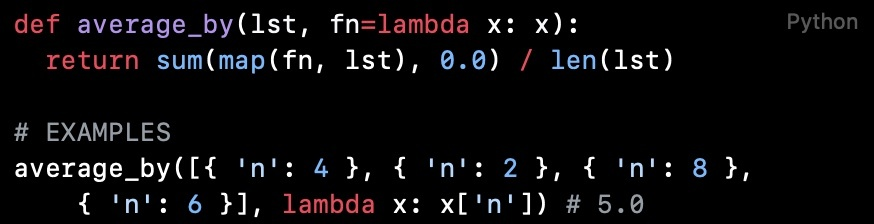# Python 代码阅读（第 1 篇）：列表映射后的平均值Félix## average_by

def average_by(lst, fn=lambda x: x):  return sum(map(fn, lst), 0.0) / len(lst)# EXAMPLESaverage_by([{ 'n': 4 }, { 'n': 2 }, { 'n': 8 }, { 'n': 6 }], lambda x: x['n']) # 5.0

### map函数

map函数是 Python 内置的一个高阶函数，这个函数很有意思，它的参数是一个函数以及一个可迭代对象。它会返回一个迭代器，这个迭代器会将参数中的函数应用在参数中可迭代对象上。

## 其他类似函数

30-seconds-of-python中还有一些类似的代码片段。在理解了average_by函数之后，这些都很容理解。

### max_by

def max_by(lst, fn):  return max(map(fn, lst))# EXAMPLESmax_by([{ 'n': 4 }, { 'n': 2 }, { 'n': 8 }, { 'n': 6 }], lambda v : v['n']) # 8

### min_by

def min_by(lst, fn):  return min(map(fn, lst))# EXAMPLESmin_by([{ 'n': 4 }, { 'n': 2 }, { 'n': 8 }, { 'n': 6 }], lambda v : v['n']) # 2

### sum_by

def sum_by(lst, fn):  return sum(map(fn, lst))# EXAMPLESsum_by([{ 'n': 4 }, { 'n': 2 }, { 'n': 8 }, { 'n': 6 }], lambda v : v['n']) # 20## 评论